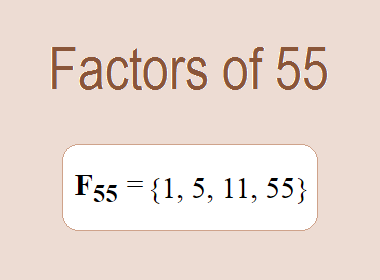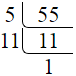# Factors of 55The factors of 55 are 1, 5, 11, and 55 i.e. F55 = {1, 5, 11, 55}. The factors of 55 are all the numbers that can divide 55 without leaving a remainder.

We can check if these numbers are factors of 55 by dividing 55 by each of them. If the result is a whole number, then the number is a factor of 55. Let's do this for each of the numbers listed above:

·        1 is a factor of 55 because 55 divided by 1 is 55.

·        5 is a factor of 55 because 55 divided by 5 is 11.

·        11 is a factor of 55 because 55 divided by 11 is 5.

·        55 is a factor of 55 because 55 divided by 55 is 1.

## How to Find Factors of 55?

1 and the number itself are the factors of every number. So, 1 and 55 are two factors of 55. To find the other factors of 55, we can start by dividing 55 by the numbers between 1 and 55. If we divide 55 by 2, we get a remainder of 1. Therefore, 2 is not a factor of 55. If we divide 55 by 3, we get a remainder of 1. Therefore, 3 is not a factor of 55.

Next, we can check if 4 is a factor of 55. If we divide 55 by 4, we get a remainder of 3. Therefore, 4 is also not a factor of 55. If we divide 55 by 5, we get a remainder of 0. Therefore, 5 is a factor of 55. We can continue this process for all the possible factors of 55.

Through this process, we can find that the factors of 55 are 1, 5, 11, and 55. These are the only numbers that can divide 55 without leaving a remainder.

********************

********************

## Properties of the Factors of 55

The factors of 55 have some interesting properties. One of the properties is that the sum of the factors of 55 is equal to 72. We can see this by adding all the factors of 55 together:

1 + 5 + 11 + 55 = 72

Another property of the factors of 55 is that the prime factors of 55 are 5 and 11.

## Applications of the Factors of 55

The factors of 55 have several applications in mathematics. One of the applications is in finding the highest common factor (HCF) of two or more numbers. The HCF is the largest factor that two or more numbers have in common. For example, to find the HCF of 55 and 65, we need to find the factors of both numbers and identify the largest factor they have in common. The factors of 55 are 1, 5, 11, and 55. The factors of 65 are 1, 5, 13, and 65. The largest factor that they have in common is 5. Therefore, the HCF of 55 and 65 is 5.

Another application of the factors of 55 is in prime factorization. Prime factorization is the process of expressing a number as the product of its prime factors. The prime factors of 55 are 5, and 11, since these are the only prime numbers that can divide 55 without leaving a remainder. Therefore, we can express 55 as:

55 = 5 × 11

We can do prime factorization by division and factor tree method also. Here is the prime factorization of 55 by division method,55 = 5 × 11

Here is the prime factorization of 55 by the factor tree method,55 = 5 × 11## Conclusion

The factors of 55 are the numbers that can divide 55 without leaving a remainder. The factors of 55 are 1, 5, 11, and 55. The factors of 55 have some interesting properties, such as having a sum of 72. The factors of 55 have several applications in mathematics, such as finding the highest common factor and prime factorization.